# Subtract Multiple Cells in Excel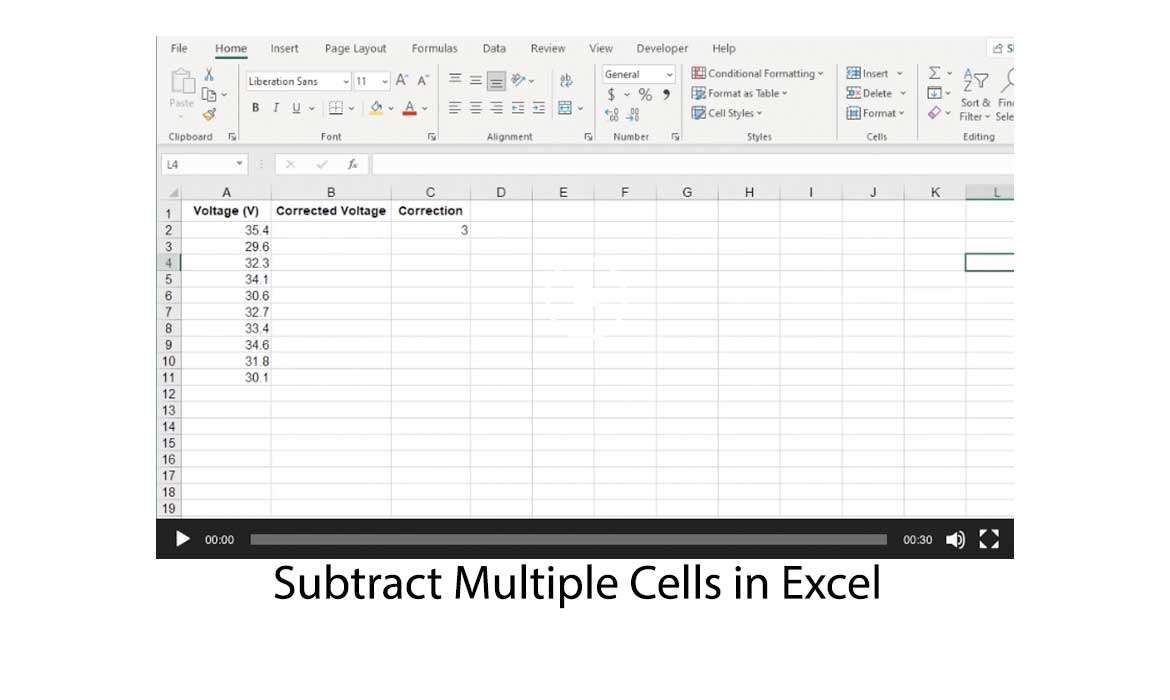Have you ever come across the situation where you have a running total, perhaps the sum of incoming payments, from which you have to subtract values from a range of cells, possibly representing expenditures?

Or perhaps you’ve had to make a correction to a series of data values such as temperatures, voltage measurements, or exam grades, by subtracting a value contained in another cell?

So, whether subtracting a value from a range or cells, or vice versa, it turns out that Excel can perform both with relative ease.

## Subtract a single value from a range of cells using a formula

In the following sheet, we have a sample of voltage measurements taken, that need to be corrected.

The correction involves subtracting 3 volts.

The original values are in column A, with the heading Voltage (V).

With such a small data set, you may think why not just do it manually.

However, sheets are rarely this small, so going through and manually updating values would be very time consuming.

Another reason, and perhaps more importantly though, is that doing this manually can be error prone.

In addition to the Voltage column, two more columns have been added.

Column B with the heading Corrected Voltage, which will contain the new, corrected voltage measurements, and column C with the heading Correction, which will have the voltage value to subtract.In the above sheet, we begin by entering the amount of correction into Cell C2.

For this example, we will use a value of 3.

This value will be subtracted from all the voltages in column A, and the corrected voltages entered into the corresponding row cell in column B.We now need to enter the following formula, =A2-\$C\$2 in cell B2.

Notice the use of the dollar symbols when referencing cell C2.

Using plain C2 is known as a relative cell reference, whereas \$C\$2 is an absolute cell reference.

We’ll discover the significance of this in a moment.Now press enter on the keyboard to set the formula.

At this stage, the new and corrected value of 32.4 will appear in cell B2.

But this has only calculated the value for a single cell.

If you move to cell B2, there is a fill handle in the bottom right hand corner of the bounding box highlighting the cell.Now grab and drag with the mouse, the fill handle down the length of column B so as to cover the corresponding voltage values contained in the cells in column A, the formula is automatically copied down each cell in column B.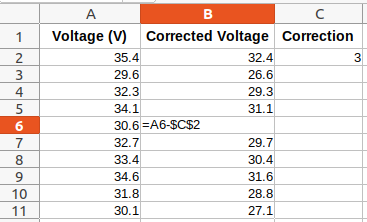Excel automatically copies the cell originally contained in B2 to the other cells in column B, updating the cell references.

For example, in the above snapshot, we see that the formula in cell B6 has been updated to =A6-\$B\$2.

Notice in the formula, that cell A2 in the original formula has been updated to A6.

However, \$B\$2 has remained the same.

This is the significance of using an absolute reference.

The reference does not update.

## Subtract a series of values from a single value

A slight variation to the above would be if we wanted to find the difference a range of voltages have from an upper limit.The range of voltages in column A are the same as before. In column C we have the Upper Limit, while the resulting differences will be output to column B.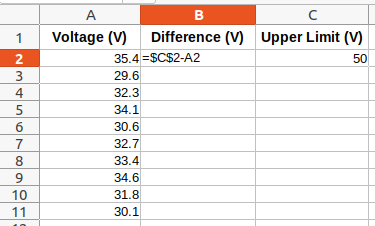We start by entering our initial formula in cell B2, =\$C\$2-A2

As before, we use absolute referencing when referring to the cell with the upper limit, and relative referencing for the cell with the voltage measurements.It is now left to drag the fill handle of the bounding box surrounding cell B2, down column B to cover the voltage values in column B.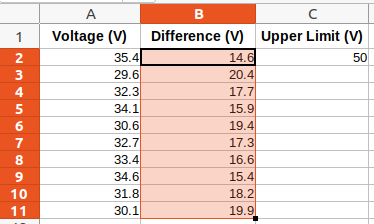The result is the list of values in column B, after subtracting the voltage in each of the cells A2 to A11, from the upper limit in cell C2.

## Subtract a single value from a range of cells without a formula

Another way to subtract a single value from a range of cells, involves using the Paste Special option in Excel.

Begin by selecting cell C2 and copying its contents.

Then select the range of cells from A2 to A11.

Right click in the selected range and from the context menu, choose Paste Special… This will open the Paste Special dialog box.

In the Operation section, choose Subtract and click the OK button.

The voltage values in Column A will now each have had subtracted, the value copied from cell C2.

One major difference between this method and the previous using a formula, is that if you wanted to change the value of the correction in cell C2, with a formula, the values in the result cells would be automatically updated.

Whereas with the Paste Special method, you would have to go through the whole process again.

## Subtract a range of cells from a single cell

For this case, we’re going to be using a simple incoming payments and expenses sheet, where we’ve summed our weekly payments and now want to subtract any expenses.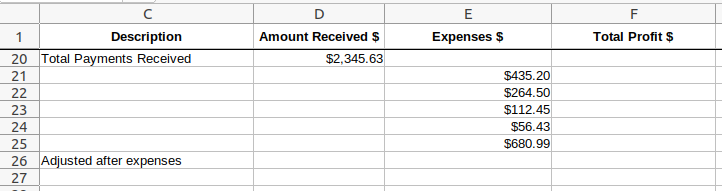The total weekly payments received are in cell D20, while the weekly expenses are in cells E21 to E25.

We want to subtract all of the expenses in column E, from the total payments received in cell D20 to produce our weekly profit, which we will store in cell F26.We enter the following formula in cell F26:

=D20-SUM(E21:E25).

The formula sums all the Expenses amounts contained in cells E21 to E25, and then subtracts that from the amount in cell D20.## Conclusion

Doing simple mathematical calculations like subtracting a value from a range of cells is quite simple and straightforward in Excel.

The same methods can be used to also add, multiply, or divide.

Furthermore, while we’ve used numerical values in our examples, you can also use dates, times, or percentages.

Excel can handle them all.

Costas has a wide range of experience in Information Technology covering computer hardware, programming, telecommunications, networking, web services, and general IT support. He's worked in various roles such as PHP programmer and web developer, technical and desktop support, hardware repair, system administration. Costas has excellent background in Microsoft Windows and Office Suite (Excel, Word, Powerpoint, etc), as well as a thorough understanding of Networking and Hardware maintenance.